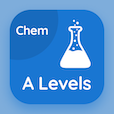Cambridge Online Courses (GCE)

A Level Chemistry Quizzes

A Level Chemistry Quiz PDF - Complete

# Chemical Formula and Equations Quiz MCQ Online p. 345

Practice Chemical Formula and Equations quiz questions and answers PDF, chemical formula and equations trivia questions 345 to learn online A Level Chemistry course for online classes. Moles and Equations MCQ questions, chemical formula and equations Multiple Choice Questions (MCQ) for online college degrees. "Chemical Formula and Equations Quiz" PDF eBook: cells and batteries, arenes reaction, reversible reactions, chemistry: nitrogen, chemical formula and equations test prep for online college for teaching degree.

"The name given to ions containing oxygen (O2) are usually called" MCQ PDF: oxides, oxalates, ates, and oxy for online colleges for science. Solve moles and equations questions and answers to improve problem solving skills for accredited online colleges.

## Trivia Quiz on Chemical Formula and Equations MCQs

MCQ: The name given to ions containing oxygen (O2) are usually called

oxalates
oxides
ates
oxy

MCQ: Plants need Nitrogen (N2) to aid in the formation of

Chlorophylls
Nitrogenous acids
Protoplast
cytoplasm

MCQ: The particular condition of equilibrium is

dynamic only
closed system only
irreversible
dynamic and closed system

MCQ: In benzene's reactions, benzene maintains its highly stable delocalized pi bonding due to

electron's intact
proton's intact
ions intact
neutron's intact

MCQ: A secondary cell used in a car battery is composed of plates of

### More Quizzes from A Level Chemistry Course